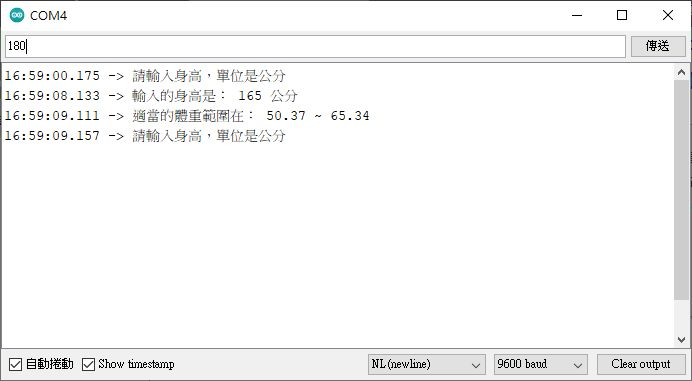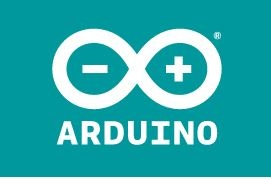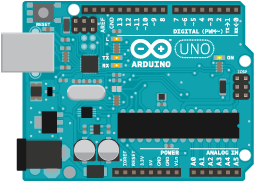## 第 02 章 -初步了解 arduino IDE 基本語法

###### 上次更新日期 2022/09/14

1. 應用 USB serial connection 方式傳輸訊息
2. 了解變數的設定與使用
3. 了解流程控制 (if) 與迴圈 (for, while) 的應用
4. 使用自訂函數的功能
5. 由序列埠讀入資料，並加以判斷使用
6. 資料型別的轉換功能

### 2.1： 了解 USB 序列埠連接訊息傳遞方式

• 使用 Serial 函數

arduino 與 IDE 開發軟體之間的傳輸，最簡單的方法當然就是透過那條原本的 USB 傳輸線！在 arduino 裡面， 可以透過已經撰寫好的 Serial (注意大小寫) 函數來處理！而一開始要處理的，就是 arduino 與 IDE 之間的傳輸速率， 或稱為鮑率 (baud rate)。想像一下，你在大隊接力的時候，最終接棒時，傳棒與接棒的人，速度應該要一致才好！ 後面的人太快就會撞在一起，前面的人太快就會無法接棒！所以在接棒區，應該速度要一致！同樣的道理， 你的 arduino 硬體與 IDE 軟體之間傳輸的速率 (鮑率) 也要相同，否則傳輸就會出問題！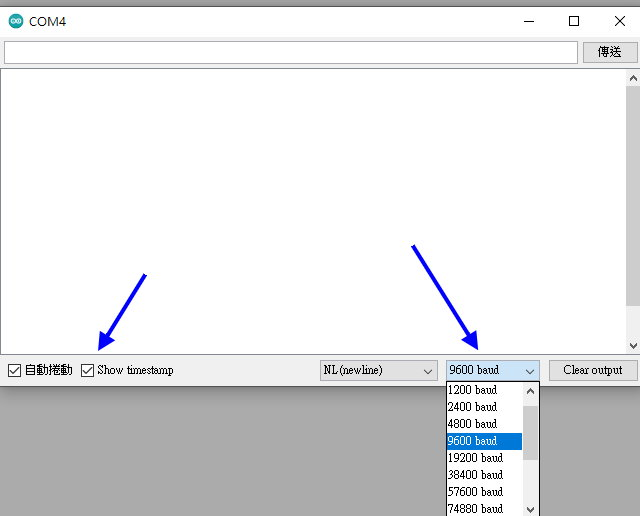```void setup() {
Serial.begin(9600);
}
void loop() {
}
```

• Serial.print(資料);
可以是字串("字串")、數值(38.38)、十進位轉為二進位的數值(38,BIN)，這個資料之間是相連的，不會主動斷行， 斷行可以加 ("\n") 處理。至於字串資料，建議使用雙引號來處理！
• Serial.println(資料);
與 Serial.print 類似，只是資料結尾會主動加上斷行字元而已
• Serial.write(資料);
可以直接輸出字串 ("字串")，如果填寫數值時，則會輸出該數值代表的 ASCII 符號！

```void setup() {
Serial.begin(9600);
}
void loop() {
Serial.print('hello Arduino\n');
delay(2000);
}
```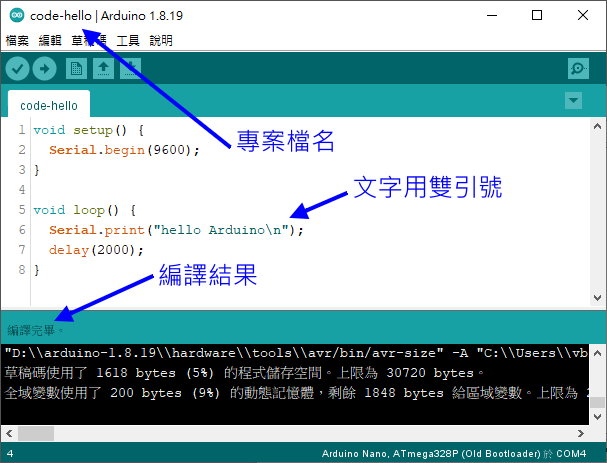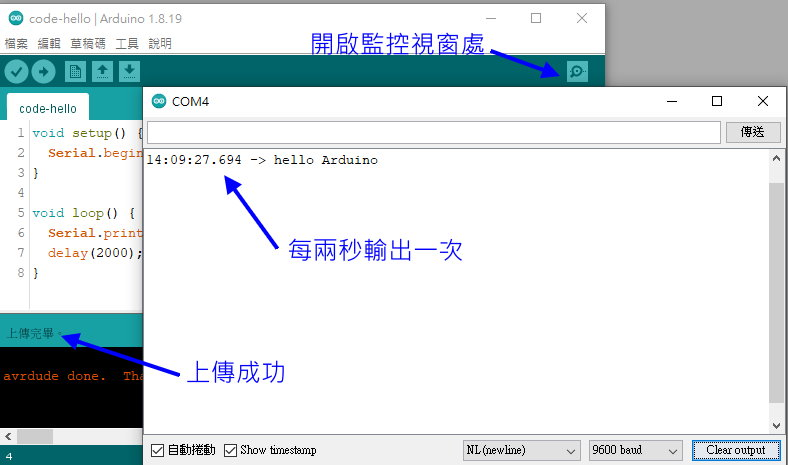### 2.2： 變數的宣告與運算子

 資料型態 佔用位元數 資料範圍 boolean (布林值) 8 true(定義!=0), false(定義=0) char (字元) 8 -128~+128 unsigned char (不帶符號字元) 8 0~255(沒有前導字元的符號) int (整數) 16 -32768~+32768 unsigned int (不帶符號整數) 16 0~65535 long (長整數) 32 -2147483648~+2147483648 float (小數,浮點數) 32 -3.4028235E+38~+3.4028235E+38 double (倍精數) 32 -3.4028235E+38~+3.4028235E+38 String (字串) 不固定 得要雙引號 "字串" 來引用
• char 字元、字串變數的宣告

```char str1 = 'a';            // 宣告為字元 a
char str2[] = "arduino";    // 宣告為一個字串 arduino
String str3 = "ARDUINO";    // 直接宣告為字串 ARDUINO
```

```void setup() {
Serial.begin(9600);
}

void loop() {
char str1   = "arduino";
char str2[] = "arduino";
String str3 = "ARDUINO";
Serial.print("str1 = ");
Serial.println(str1);
Serial.print("str2 = ");
Serial.println(str2);
Serial.print("str3 = ");
Serial.println(str3);
delay(2000);
}
```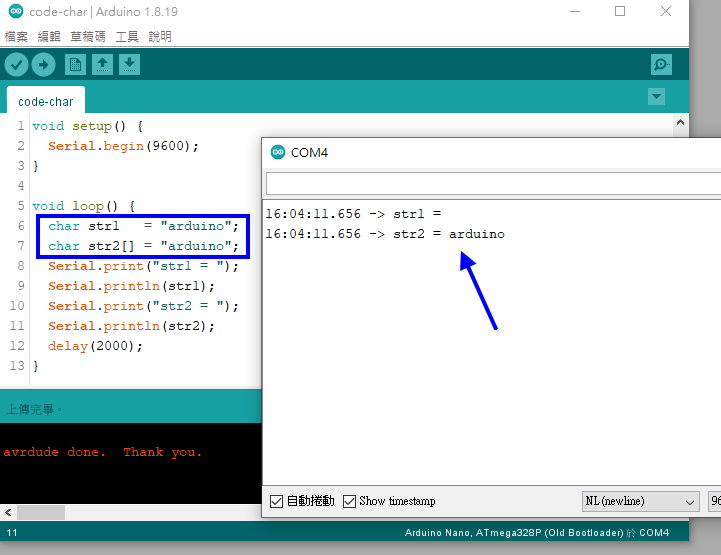• 常見宣告方式

```  int myvar = 100;                   // 宣告一個整數，且數值為 100
int myi, myj, myk;                 // 宣告三個整數變數，不給初始值
float myf1 = 3.14159;              // 宣告一個浮點數，並給予數值 3.14159
String mystr[] = "hello arduino";  // 宣告一個字串變數，內容為 hello arduino
```
• 算術運算子

 運算子 行為 範本 說明 + 加法 x+y 兩者相加 - 減法 x-y 兩者相減 * 乘法 x*y 兩者相乘 / 除法 x/y 兩者相除 % 整數取餘數 x%y x除以y的餘數 ++ 遞增 x++ 相當於 x=x+1 -- 遞減 x-- 相當於 x=x-1

```void setup() {
Serial.begin(9600);
}

void loop() {
float V;
float r = 5.0;
V = 4/3*3.14159*r*r*r;
Serial.print("半徑為");
Serial.print(r);
Serial.print("的球體的體積是： ");
Serial.println(V);
delay(2000);
}
```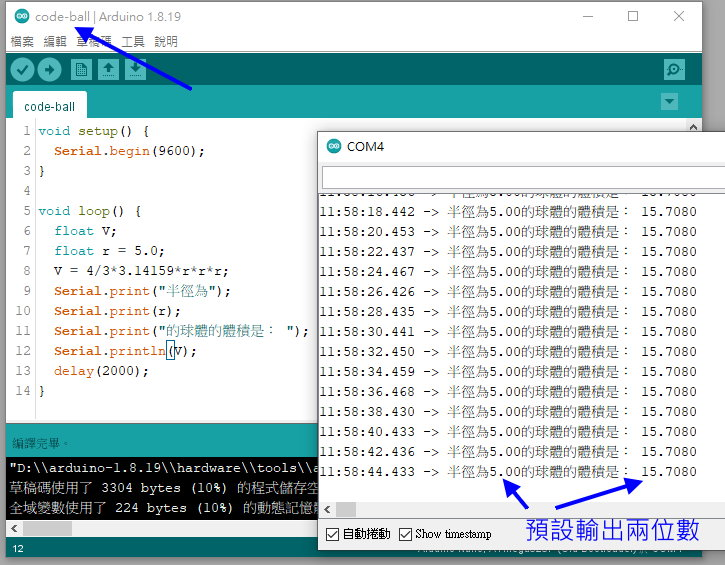```  Serial.println(V, 4);   // 代表浮點數後面接 4 個小數點位數！
```

• 變數型態轉換、全域與區域變數

```  String(var);
char(var);
var.toInt()
var.toFloat();
```

```int mypi = 3.14;
void setup() {
....
}
void loop() {
char mystr[] = 'arduino';
....
float Q = mypi * r * r;
}
void fun() {
....
}
```

• 關係運算子

 運算子 行為 範本 說明 == 等於 x==y 若 x 與 y 相同，則為 true，否則是 false != 不等於 x!=y 若 x 與 y 不相同，則為 true，否則是 false > 大於 x>y 若 x 大於 y ，則為 true，否則是 false < 小於 x= 大於等於 x>=y 若 x 大於等於 y ，則為 true，否則是 false <= 小於小於 x<=y 若 x 小於等於 y ，則為 true，否則是 false

• 數值運算與輸出

```void setup() {
Serial.begin(9600);
}

void loop() {
float myh = 1.76;
float myw = 68.0;
float mybmi = myw / myh / myh;
Serial.print("身高： ");
Serial.println(myh);
Serial.print("體重： ");
Serial.println(myw);
Serial.print("BMI： ");
Serial.println(mybmi);
delay(2000);
}
```

### 2.3： 流程控制 if 與 switch

```void setup() {
....
}

void loop() {
if ( 條件判斷 ) {
....
}
}
```

```void setup() {
....
}

void loop() {
if ( 條件判斷 )
....
}
```

 運算子 行為 範本 說明 && and x==y && a==b 兩者皆為 true 才是 true，否則是 false || or x==y || a==b 其中一個是 true 就是 true，否則是 false

```void setup() {
Serial.begin(9600);
}

void loop() {
float myh = 1.76;
float myw = 68.0;
float mybmi = myw / myh / myh;
String mycheck = "體重不是很正常喔！";
if ( mybmi > 18.5 && mybmi < 24 )
mycheck = "體重正常" ;
Serial.print("身高： ");
Serial.println(myh);
Serial.print("體重： ");
Serial.println(myw);
Serial.print("BMI： ");
Serial.println(mybmi);
Serial.print("體重狀況： ");
Serial.println(mycheck);
delay(2000);
}
```

```void loop() {
if (條件判斷) {
....
} else if {
....
} else {
....
}
}
```

• BMI < 18.5 ：過輕
• 18.5 < BMI < 24 ：正常！
• 24 < BMI < 27 ：偏重
• 27 < BMI < 30 ：輕度肥胖
• 30 < BMI < 35 ：中度肥胖
• BMI > 35 ：重度肥胖
```  String mycheck ;
if ( mybmi < 18.5 ) {
mycheck = "有點太輕";
} else if ( mybmi < 24 ) {
mycheck = "體重狀況不錯";
} else if ( mybmi < 27 ) {
mycheck = "有點偏重，注意飲食";
} else if ( mybmi < 30 ) {
mycheck = "輕度肥胖";
} else if ( mybmi < 35 ) {
mycheck = "中度肥胖";
} else if ( mybmi >= 35 ) {
mycheck = "重度肥胖";
}
```
• 使用 switch 固定變數內容

```switch (變數名稱) {
case 變數值-1 : {
....;
}
break;
case 變數值-2 : {
....;
}
break;
....
default: {
....;
}
}
```

```void loop() {
int mypw = 3;  // 分別帶入 0, 1, 2 再上傳看看
String mycheck;
switch (mypw) {
case 1: {
mycheck = "有電有電！是 ON 的！";
}
break;
case 0: {
mycheck = "停電狀態是 OFF 啦";
}
break;
default: {
mycheck = "目前是莫名其妙的狀態";
}
}
Serial.println("狀態是： " + mycheck);
delay(2000);
}
```

### 2.4： 迴圈控制

```for (起始值; 結束條件; 數值步階) {
....
}
```

```void loop() {
int i, j;
String out;
for ( i=1; i<=9; i++ ) {
for ( j=1; j<=9; j++ ) {
out = String(i) + " * " + String(j) + " = " + String(i*j);
Serial.println(out);
}
delay(1000);
}
delay(2000);
}
```

• 使用 while 進行迴圈

while 迴圈的使用方式有點像這樣：

```while (條件判斷) {
....
}
```

```void loop() {
// put your main code here, to run repeatedly:
Serial.println("開始計算體力、魔力與戰鬥力");
int myhp, mymp, mypw;
bool check = true;
while (check) {
myhp = random(10,50);
mymp = random(10,50);
mypw = 100 - myhp - mymp;
if (mypw > 10 && mypw < 50)
check = false;  // 計算成功，就離開 while 迴圈囉！
}
Serial.println("體力值： " + String(myhp));
Serial.println("魔力值： " + String(mymp));
Serial.println("戰鬥力： " + String(mypw));
delay(5000);
}
```
• 使用 do while 迴圈

```do {
....
....
} while (條件判斷)
```

### 2.5： 自訂函數

```數值型態 函數名稱(外帶參數){ .... }

int sum(int x, int y) {
....
}
```

• 假設球體的半徑為 r
• 球體的週長會是： 2πr
• 球體的表面積會是： 4πr2
• 球體的體積會是： (4/3)πr3

```float pi = 3.14159;

void setup() {
Serial.begin(9600);
}

void loop() {
float r = 6371.0;
float res1 = perimeter(r);
float res2 = surface(r);
float res3 = volume(r);
Serial.println("地球平均週長： " + String(res1));
Serial.println("地球平均表面積： " + String(res2));
Serial.println("地球平均體積： " + String(res3));
delay(5000);
}

float perimeter(float r) {
float res = 2 * pi * r;
return(res);
}
float surface(float r) {
float res = 4 * pi * r * r ;
return(res);
}
float volume(float r) {
float res = (4/3) * pi * r * r * r;
return(res);
}
```

### 2.6： 陣列

```int x = { 1, 2, 3, 4, 5};
// x = 1;
// x = 2;
// x = 5;
```

```float pi = 3.14159;
float res;

void setup() {
Serial.begin(9600);
}

void loop() {
float r = 6371.0;
earth(r);
Serial.println("地球平均週長： " + String(res));
Serial.println("地球平均表面積： " + String(res));
Serial.println("地球平均體積： " + String(res));
delay(5000);
}

float *earth(float r) {
res = 2 * pi * r;
res = 4 * pi * r * r ;
res = (4/3) * pi * r * r * r;
return res;
}
```

```int array = { {...}, {...}, {...} }
```

### 2.7： 跟序列埠互動

從序列埠讀入資料，資料型態為字串喔！
• str.trim() 將字串 str 的前後空白刪除！

```void setup() {
Serial.begin(9600);
}

void loop() {
mystr.trim();
if ( mystr != "" ) {
Serial.println("讀到的字串是 ==> " + mystr);
}
delay(2000);
}
```

```void setup() {
Serial.begin(9600);
}

void loop() {

int myh = 0;
Serial.println("請輸入身高，單位是公分");
while ( myh <= 0 || myh >= 300 ) {
mystr.trim();
if ( mystr != "" ) {
Serial.println("輸入的身高是： " + mystr + " 公分");
myh = mystr.toInt();
}
delay(1000);
}

float myw = 0;
Serial.println("請輸入體重，單位是公斤");
while ( myw <= 0 || myw >= 300 ) {
mystr.trim();
if ( mystr != "" ) {
Serial.println("輸入的體重是： " + mystr + " 公斤");
myw = mystr.toInt();
}
}

float mybmi = myw / myh / myh * 10000;
Serial.println("你的 BMI 是：" + String(mybmi));
checkbmi(mybmi);
delay(5000);
}

void checkbmi(float mybmi) {
String mycheck ;
if ( mybmi < 18.5 ) {
mycheck = "有點太輕";
} else if ( mybmi < 24 ) {
mycheck = "體重狀況不錯";
} else if ( mybmi < 27 ) {
mycheck = "有點偏重，注意飲食";
} else if ( mybmi < 30 ) {
mycheck = "輕度肥胖";
} else if ( mybmi < 35 ) {
mycheck = "中度肥胖";
} else if ( mybmi >= 35 ) {
mycheck = "重度肥胖";
}
Serial.println("體重狀況： " + mycheck );
return;
}

```

### 2.8： 課後作業